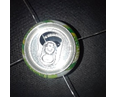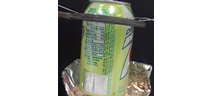# Experiment_623 Estimating the Calorie Content of Wax 1_3

$$\newcommand{\vecs}{\overset { \rightharpoonup} {\mathbf{#1}} }$$ $$\newcommand{\vecd}{\overset{-\!-\!\rightharpoonup}{\vphantom{a}\smash {#1}}}$$$$\newcommand{\id}{\mathrm{id}}$$ $$\newcommand{\Span}{\mathrm{span}}$$ $$\newcommand{\kernel}{\mathrm{null}\,}$$ $$\newcommand{\range}{\mathrm{range}\,}$$ $$\newcommand{\RealPart}{\mathrm{Re}}$$ $$\newcommand{\ImaginaryPart}{\mathrm{Im}}$$ $$\newcommand{\Argument}{\mathrm{Arg}}$$ $$\newcommand{\norm}{\| #1 \|}$$ $$\newcommand{\inner}{\langle #1, #2 \rangle}$$ $$\newcommand{\Span}{\mathrm{span}}$$ $$\newcommand{\id}{\mathrm{id}}$$ $$\newcommand{\Span}{\mathrm{span}}$$ $$\newcommand{\kernel}{\mathrm{null}\,}$$ $$\newcommand{\range}{\mathrm{range}\,}$$ $$\newcommand{\RealPart}{\mathrm{Re}}$$ $$\newcommand{\ImaginaryPart}{\mathrm{Im}}$$ $$\newcommand{\Argument}{\mathrm{Arg}}$$ $$\newcommand{\norm}{\| #1 \|}$$ $$\newcommand{\inner}{\langle #1, #2 \rangle}$$ $$\newcommand{\Span}{\mathrm{span}}$$$$\newcommand{\AA}{\unicode[.8,0]{x212B}}$$

 Student Name Laboratory Date:  Date Report Submitted: ___________________________ Student ID Experiment Number and Title Experiment 623:  Estimating the Calorie Content of Wax

Experiment 623:  Estimating the Calorie Content of Wax

Section 1:  Purpose and Summary

• Understand the general chemical formula for wax

• Set up an apparatus to measure the amount of heat generated from combustion of wax

• Calculate the calorie content of wax

Alkanes are saturated hydrocarbons. They contain only carbon and hydrogen and have no multiple (double or triple) bonds present.  Low molecular weight hydrocarbons are gases like methane and ethane.  Medium molecular weight hydrocarbons are found in liquids like gasoline or diesel fuels.  High molecular weight hydrocarbons are found in tar and waxes.

When hydrocarbons are burned with oxygen in a combustion reaction, the reaction releases heat.  A general reaction equation is:

2CnH2n+2     +     (3n+1) O2      →    2n CO2     +     (2n +2) H2

Reactants Products

From the standard heats of formation of the substances involved, the heat of any reaction (ΔHoreaction) can be determined from the equation:

ΔHoreaction = ∑ ΔHof, products - ∑ ΔHof, reactants

The ΔHof (heats of formation) from published tables (NIST Webbook, CRC Handbook of Chemistry and Physics, and the like) can be used for this calculation. Note that when the ΔHoreaction is positive, heat is absorbed, and when the ΔHoreaction is negative, heat is released.

Several different sets of units can be used to measure ΔHreaction.  We will be using calories per gram and calories per mole.  “Nutritional calories” that are declared on food packaging are kilocalories (kcal), or 1000 calories.  So a candy bar that is listed as 240 calories means: 240,000 calories of energy are available by metabolizing it.

Candle wax has carbon chains that are very long, generally at least 20 or 30 carbons bound together in long chains.   For this combustion, if a value of n = 25 is used, the balanced equation becomes:

C25H52     +     38 O2     →     25 CO2     +     26 H2

In this experiment, students will use a measured amount of water to absorb the heat from a lighted candle.  By determining the increase in temperature of the water, students can calculate how much heat has been absorbed by the water and therefore, how much has been released from burning the candle.

If we assume that the amount of heat energy, q, from combustion is transferred from the flame of the candle through the aluminum can and into the water suspended above the candle, then we can describe it by the following relationship:

heat absorbed by the water  heat given off by the candle

(mass)(specific heat)(change in temperature)

q = m × c × ΔT

Be careful with your units and unit conversions.  Heat is usually given in either calories or joules, mass is in grams, the specific heat is in calories per gram degree C, and change in temperature is in degrees C.

If the candle is weighed before and after heating, students can measure the mass lost during the combustion.  This will allow for the calculation of the heat generated per unit mass of candle wax combusted.

Section 2:  Safety Precautions and Waste Disposal

Safety Precautions:

Use of eye protection is recommended for all experimental procedures.

Waste Disposal:

The aluminum cans be reused. The burned candle can be disposed of in the garbage bin.

Section 3: ProcedureObtain a clean, empty, dry aluminum can.  Measure the mass of the can to the nearest 0.01 g and record this value.  Using a thumbtack, pierce the can about 1/3 height of the can in four spots.  Open up a large paperclip and run the paperclip through the can from one side to the other.  Repeat with a second paperclip.  You should now be able to suspend the can from an iron ring by the paperclips.  See the photo to the left. Mass of aluminum can:    _______________ grams Place the can on a balance that measures to the nearest 0.01 g.  Tare the balance.  Add approximately 100 ml of laboratory water to the can and measure the mass of water added.  Record this value. Mass of aluminum can and laboratory water:     _______________ grams Obtain a candle and measure its mass to the nearest 0.001 g. Initial mass of candle:    _______________ grams Measure the initial temperature of the water in the can to the nearest 0.1 degree Celsius.  Either a glass themometer or a digital thermometer may be used. Initial temperature of the water:     _______________ oCPlace the candle under the can.  Wrap with aluminum foil the lower half of the can and the space between the can and the base of the candle around the can (see right).  (The candle will not remain lit if you completely surround the space.)  Light the candle and heat the can directly with the flame.  Monitor the temperature until there is an increase in the temperature of the water of 10 degrees Celsius.  This should take less than 10 minutes. Extinguish the candle and record the final temperature of the water in the can. Final temperature of the water:    _______________ oC Measure the final mass of the candle to the nearest 0.001g. Final mass of candle:    _______________ grams Repeat this procedure for a second measurement. If time permits, perform a third measurement. Tabulate your data in the worksheet below.

Section 4: Calculations and Tabulation of Data

 Calculate the mass of the laboratory water in the can:  [(b) – (a)], in grams. Mass of water (g): Calculate the change in temperature of the water, ΔT = Tf - Ti,  [(e) – (d)] ΔT (oC): Using the specific heat of water, 1.00 cal/g-oC, calculate the amount of heat absorbed by the water from the burning candle. Amount of heat absorbed by the water, (cal): Calculate the mass of the burned candle: [(f) – (c)] Mass of burned candle (g): Calculate the calorie content of the wax from this candle, in cal/g. Make sure to include the appropriate sign (+ or -). Calorie content of wax, cal/g: Determine the average calorie content of wax from your result and compare with the class.

Data Summary:

 Trial 1 Trial 2 Trial 3 Mass of water, g Change of temperature of water, oC Amount of heat absorbed by the water, cal Mass of burned candle, g Calorie content of wax, cal/g Average calorie content of wax, cal/g Average value for the class

Post Lab Questions:

1. Many tables use values of joules per mole, or kilojoules per mole.  Using a chemical formula of C25H52 for wax, convert your value of the calorie content per gram into the units of calories per mole, kilocalories per mole and kilojoules per mole.

1. Using thermodynamic data available, calculate the molar heat of combustion (ΔHocomb ) for the following chemical equation:

C25H52     +     38 O2     →     25 CO2     +     26 H2

1. How does the class average calorie content of wax compare to the calculated value above (2).

1. Aluminum has a specific heat of 0.215 cal / g °C .  Calculate the amount of heat that is absorbed by the can for one of your trials.  Is this a significant amount relative to the amount of heat absorbed by the water?  If it is, correct your three trials.

Notes:

Experiment_623 Estimating the Calorie Content of Wax 1_3 is shared under a CC BY license and was authored, remixed, and/or curated by LibreTexts.